Divisible

Chapter 4 Class 11 Mathematical Induction
Concept wise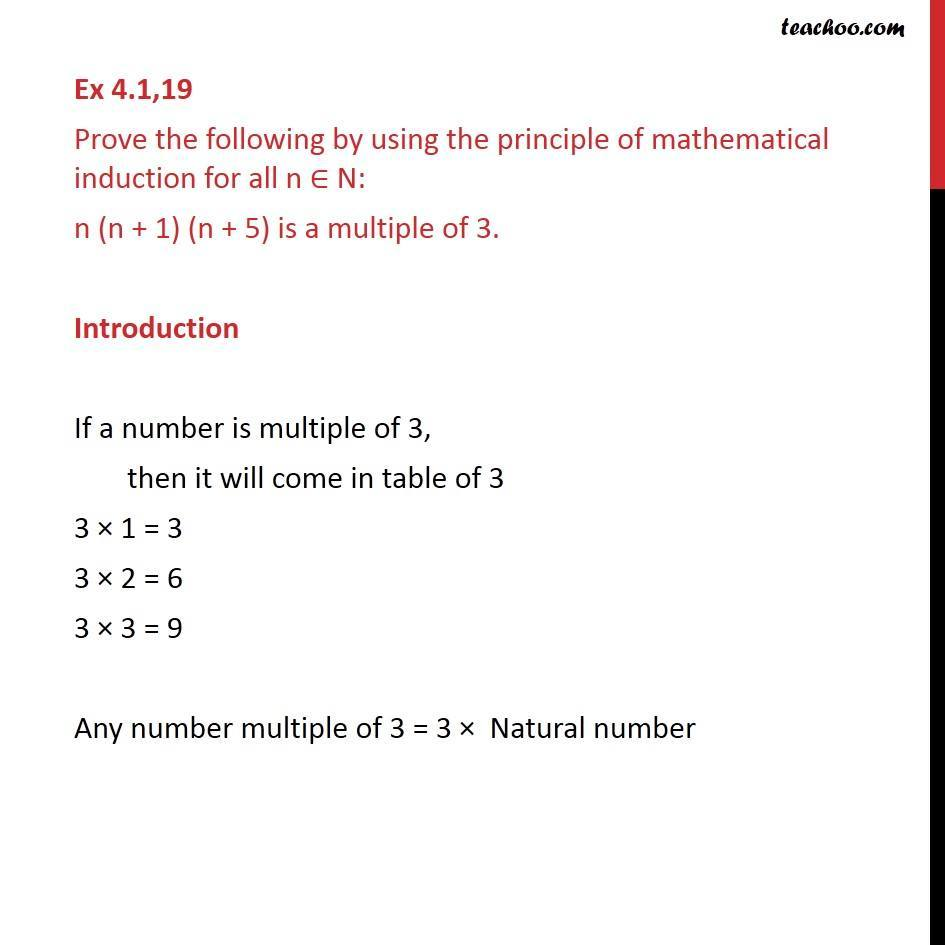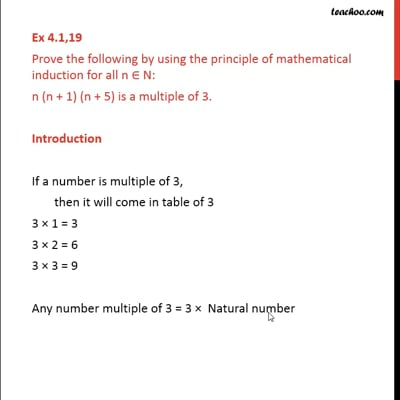This video is only available for Teachoo black users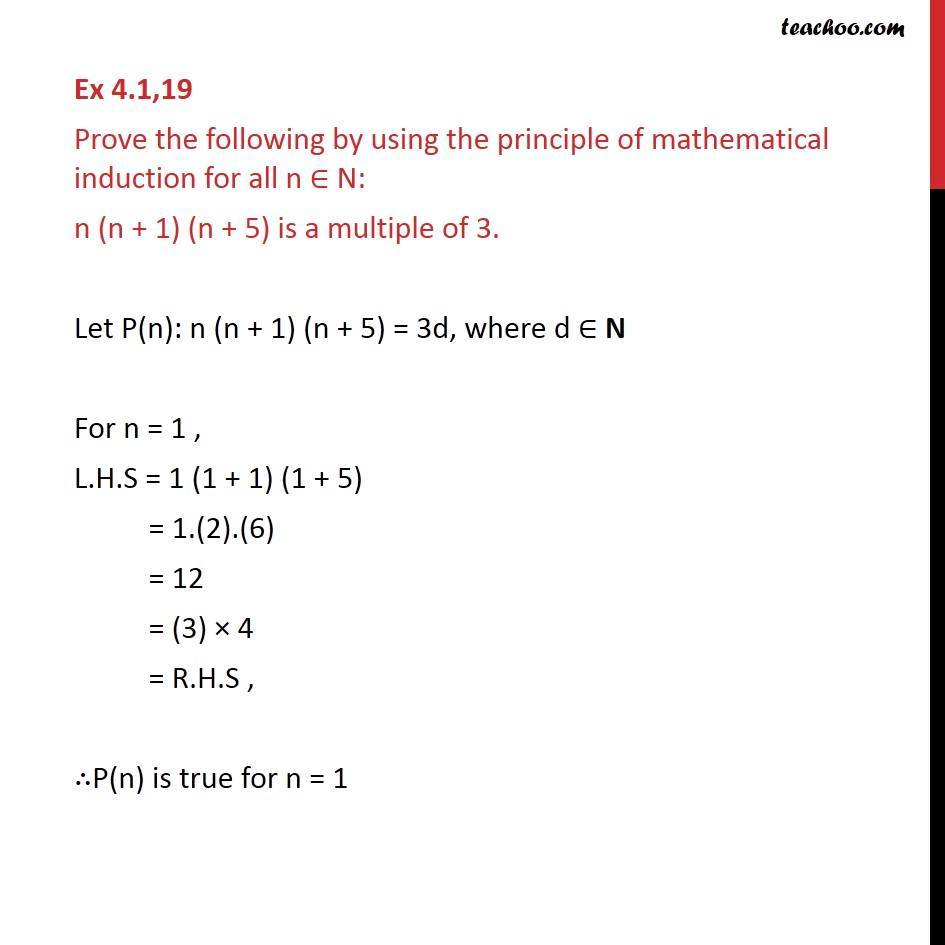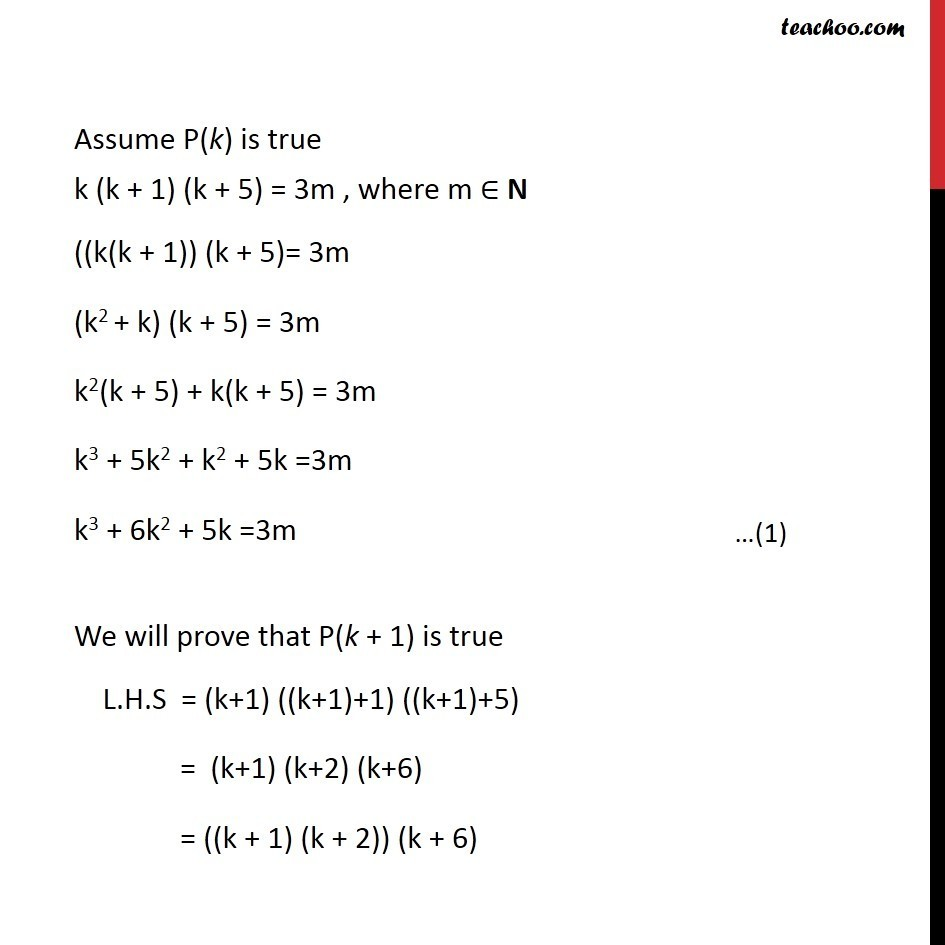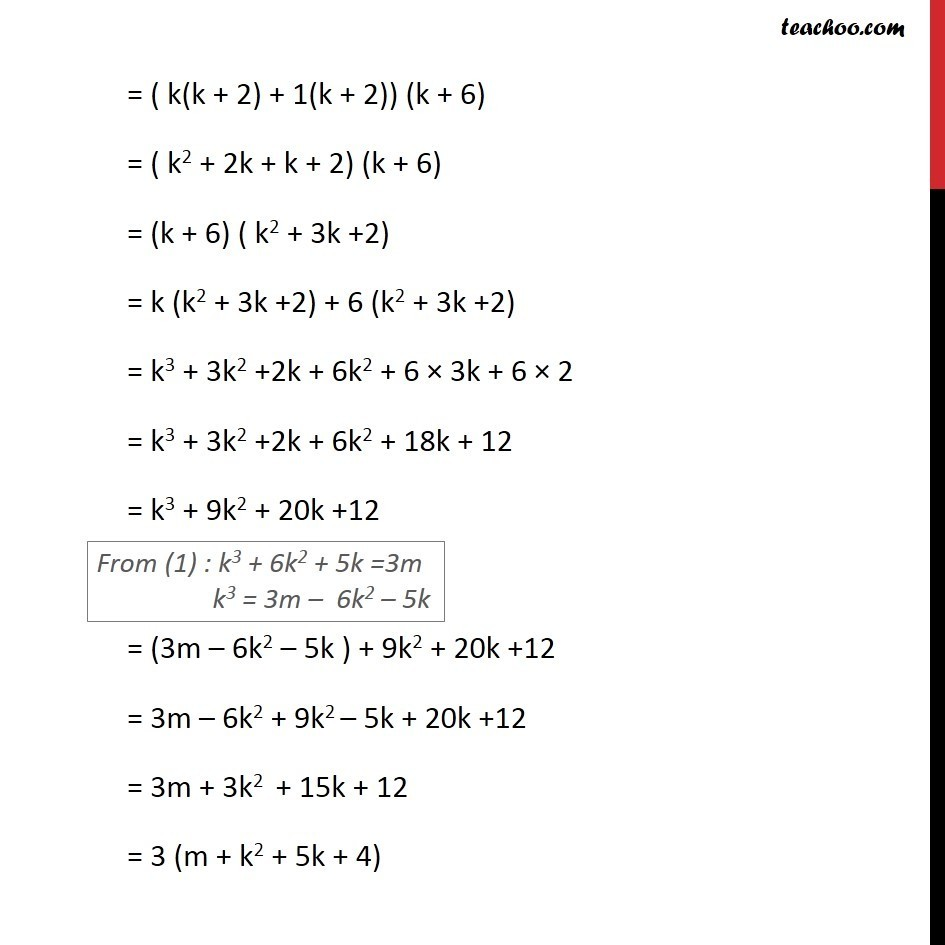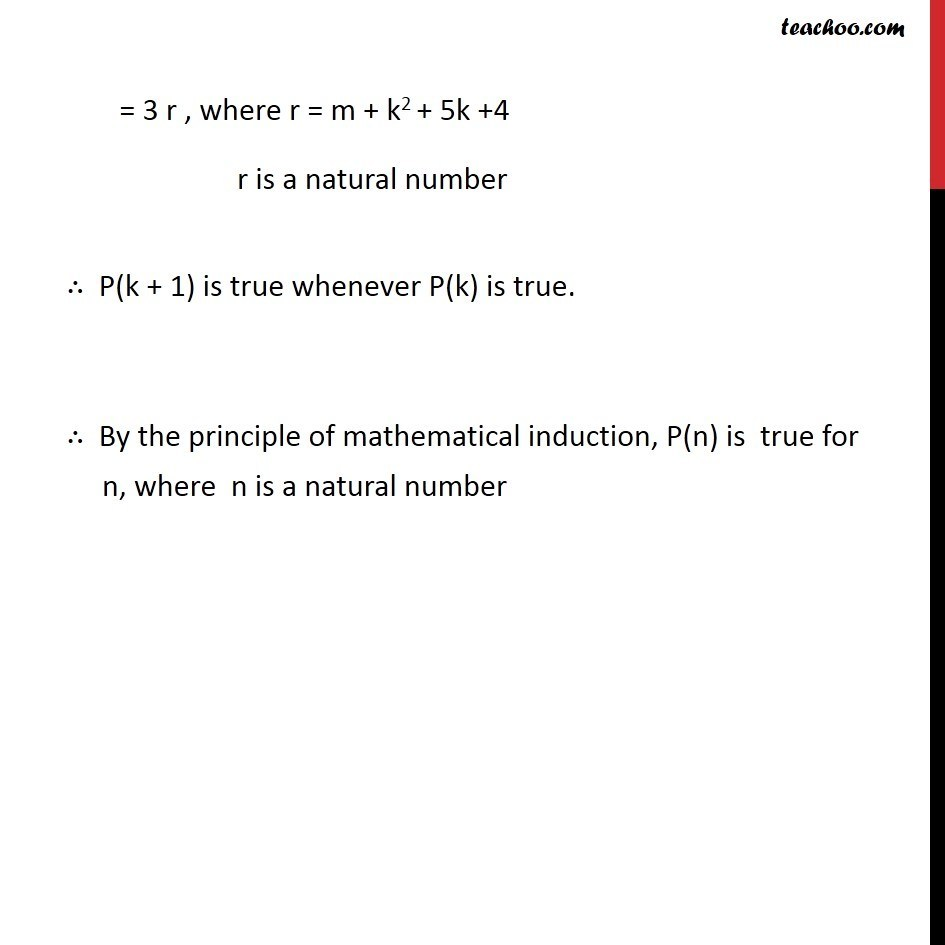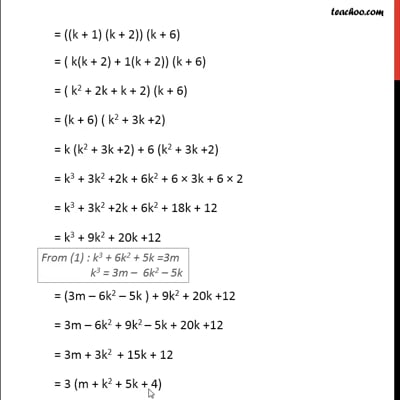This video is only available for Teachoo black users

Introducing your new favourite teacher - Teachoo Black, at only ₹83 per month

### Transcript

Ex 4.1,19 Prove the following by using the principle of mathematical induction for all n ∈ N: n (n + 1) (n + 5) is a multiple of 3. Introduction If a number is multiple of 3, then it will come in table of 3 3 × 1 = 3 3 × 2 = 6 3 × 3 = 9 Any number multiple of 3 = 3 × Natural number Ex 4.1,19 Prove the following by using the principle of mathematical induction for all n ∈ N: n (n + 1) (n + 5) is a multiple of 3. Let P(n): n (n + 1) (n + 5) = 3d, where d ∈ N For n = 1 , L.H.S = 1 (1 + 1) (1 + 5) = 1.(2).(6) = 12 = (3) × 4 = R.H.S , ∴P(n) is true for n = 1 Assume P(k) is true k (k + 1) (k + 5) = 3m , where m ∈ N ((k(k + 1)) (k + 5)= 3m (k2 + k) (k + 5) = 3m k2(k + 5) + k(k + 5) = 3m k3 + 5k2 + k2 + 5k =3m k3 + 6k2 + 5k =3m We will prove that P(k + 1) is true L.H.S = (k+1) ((k+1)+1) ((k+1)+5) = (k+1) (k+2) (k+6) = ((k + 1) (k + 2)) (k + 6) = ( k(k + 2) + 1(k + 2)) (k + 6) = ( k2 + 2k + k + 2) (k + 6) = (k + 6) ( k2 + 3k +2) = k (k2 + 3k +2) + 6 (k2 + 3k +2) = k3 + 3k2 +2k + 6k2 + 6 × 3k + 6 × 2 = k3 + 3k2 +2k + 6k2 + 18k + 12 = k3 + 9k2 + 20k +12 = (3m – 6k2 – 5k ) + 9k2 + 20k +12 = 3m – 6k2 + 9k2 – 5k + 20k +12 = 3m + 3k2 + 15k + 12 = 3 (m + k2 + 5k + 4) = 3 r , where r = m + k2 + 5k +4 r is a natural number ∴ P(k + 1) is true whenever P(k) is true. ∴ By the principle of mathematical induction, P(n) is true for n, where n is a natural number